Home | | Maths 10th Std | Multiple choice questions

# Multiple choice questions

Mathematics : Algebra: Multiple choice questions with answers / choose the correct answer with answers - Maths Book back 1 mark questions and answers with solution for Exercise Problems

## Multiple choice questions

1. A system of three linear equations in three variables is inconsistent if their planes

(1) intersect only at a point

(2) intersect in a line

(3) coincides with each other

(4) do not intersect2. The solution of the system x + y − 3x = −6 ,  − 7y + 7z = 7 , 3z = 9  is

(1) x = 1, y = 2, z = 3

(2) x = −1, y = 2, z = 3

(3) x = − 1, y = −2, z = 3

(4) x = 1, y = 2, z = 33. If (x - 6) is the HCF of x2 - 2x - 24 and x2 - kx - 6 then the value of k is

(1) 3

(2) 5

(3) 6

(4) 8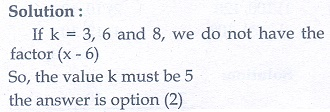4.isAns: (1)5.is not equal toAns: (2)6.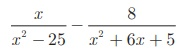givesAns: (3)7. The square root of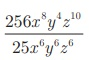is equal toAns: (4)8. Which of the following should be added to make x4 + 64 a perfect square

(1) 4x2

(2) 16x2

(3) 8x2

(4) -8x29. The solution of  2x − 1 2  = 9 is equal to

(1) -1

(2) 2

(3) –1, 2

(4) None of these10. The values of a and b if 4x 4 − 24x 3 + 76x 2 + ax +b is a perfect square are

(1) 100,120

(2) 10,12

(3) -120 ,100

(4) 12,1011. If the roots of the equation q2x2 + p2x + r2  = 0 are the squares of the roots of the equation qx2 + px + r = 0 , then q, p, r  are in _____

(1) A.P

(2) G.P

(3) Both A.P and G.P

(4) none of these12. Graph of a linear polynomial is a

(1) straight line

(2) circle

(3) parabola

(4) hyperbola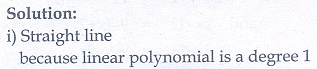13. The number of points of intersection of the quadratic polynomial x2 + 4x + 4 with the X axis is

(1) 0

(2) 1

(3) 0 or 1

(4) 2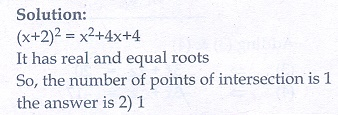14. For the given matrix A =the order of the matrix AT is

(1) 2 ×3

(2) 3 ×2

(3) 3 ×4

(4) 4 ×315. If A is a 2 ×3 matrix and B is a 3 ×4 matrix, how many columns does AB have

(1) 3

(2) 4

(3) 2

(4) 516. If number of columns and rows are not equal in a matrix then it is said to be a

(1) diagonal matrix

(2) rectangular matrix

(3) square matrix

(4) identity matrix

17. Transpose of a column matrix is

(1) unit matrix

(2) diagonal matrix

(3) column matrix

(4) row matrix

18. Find the matrix X if 2X +Ans: (2)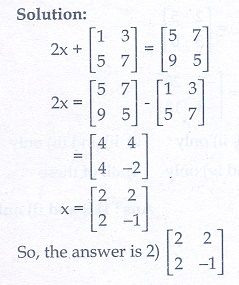19. Which of the following can be calculated from the given matrices, (i) A2 (ii) B2 (iii) AB (iv) BA

(1) (i) and (ii) only

(2) (ii) and (iii) only

(3) (ii) and (iv) only

(4) all of these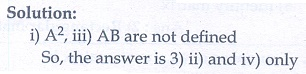20. If. Which of the following statements are correct?(1) (i) and (ii) only

(2) (ii) and (iii) only

(3) (iii) and (iv) only

(4) all of these